Next: Structure of the Galaxy Up: Model of the Galaxy Previous: Model of the Galaxy

### Frequency distribution of galactic binaries

As discussed above (SectionĀ6), galactic binary systems are assumed to be distributed logarithmically along their semi-major axis A (Abt and Levy, 1976),

Ā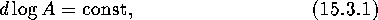with minimal and maximal separations ranging from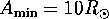to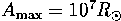. Accordingly, the initial frequency range covered by gravitational waves emitted by these binaries,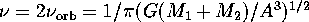, spans fromtoĀHz.

Emission of gravitational waves makes the system shrink with time (on average), so that the ``blue'' end of the initial frequency distribution expands to higher frequencies. Theoretically, it can reach as high frequency as1 kHz, corresponding to binary NS coalescenceĀ (see Figure 40).

Ā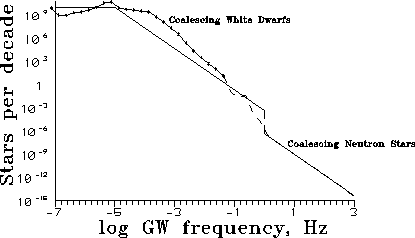Figure 40: Number of binary stars per frequency decade. The total number of stars in the Galaxy is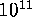. Numbers <1 are mathematical expectations. Analytical estimation (equation 15.3.5) is shown by the solid line (Lipunov et al., 1995a).Ā

As the observed distribution of binary periods is flat (equation (15.3.1)), the normalization constant is the total number of galactic binaries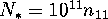per frequency decade:

Ā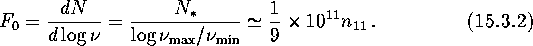It is clear that under a stationary star formation rate (which is a good approximation of the situation in our Galaxy) after some time the blue end of the GWB will be fully determined by coalescing binary white dwarfsĀ (up to1 Hz) and NS (up to1 kHz) (LPP87). The rate of frequency change for a coalescing binary is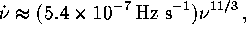where,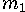,are masses of the binary components, and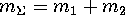expressed in solar masses. The stationary continuity equation provides us with the spectral density of the number of stars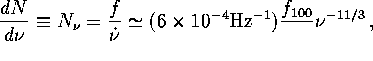where f is a coalescenceĀ rate of the binaries,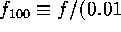Āyr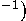. Then the number of stars per decade is

ĀThe red end of the GWB will not change and a characteristic ``break'' will appear in the spectrum. This break occurs at the frequency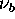defined by the matching condition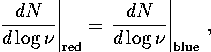therefrom one findsThis distribution will break up at a frequency of about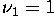ĀHz corresponding to the limiting frequency of binary WD. Only the binary NS or BH will form the blue end of the distribution up to1ĀkHz. Thus the resulting form of the distribution is:

ĀThese simple qualitative considerations fully agree with numerical calculations (see Figure 40).Next: Structure of the Galaxy Up: Model of the Galaxy Previous: Model of the Galaxy

Mike E. Prokhorov
Sat Feb 22 18:38:13 MSK 1997